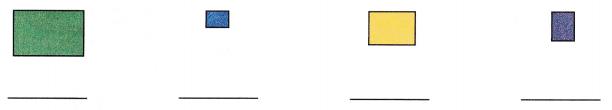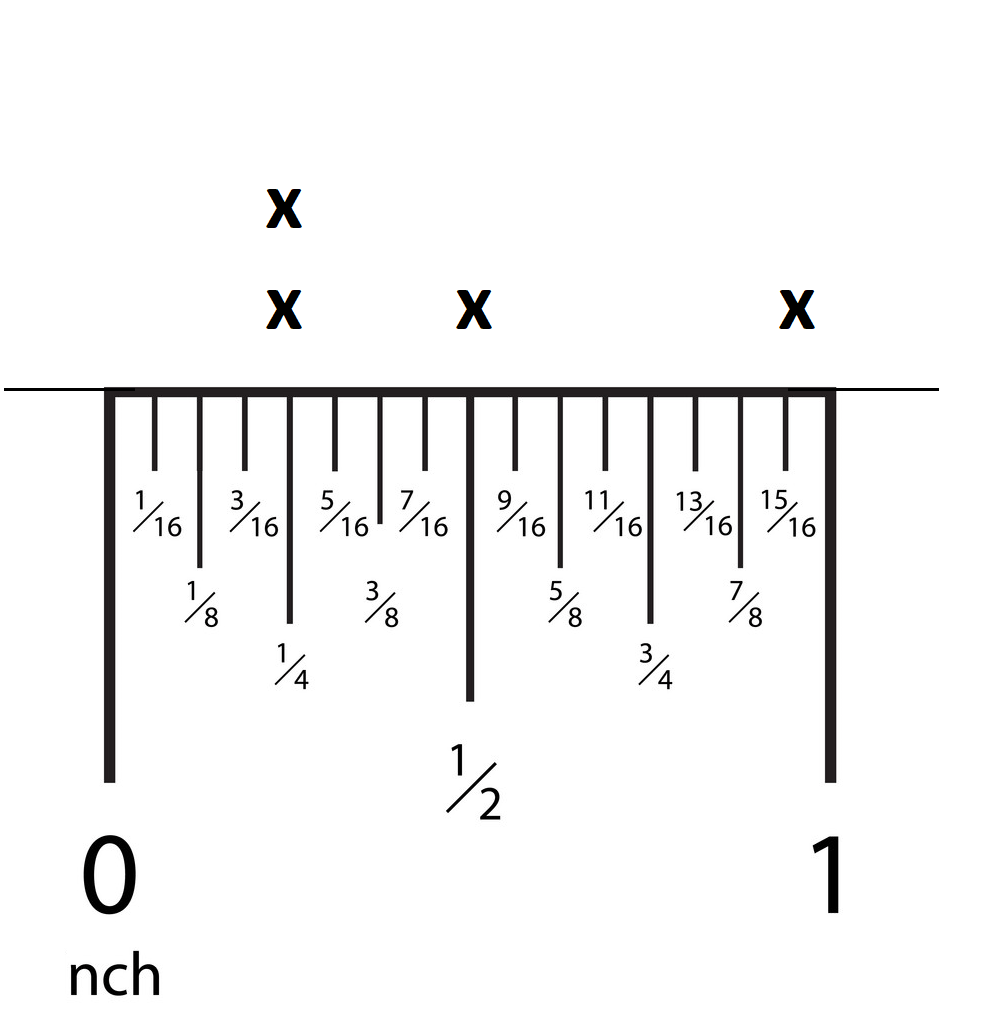# McGraw Hill Math Grade 3 Chapter 10 Lesson 7 Answer Key Drawing a Line Plot

## McGraw-Hill Math Grade 3 Answer Key Chapter 10 Lesson 7 Drawing a Line Plot

Record

Use a ruler to measure the length of the shapes to the nearest $$\frac{1}{2}$$ inch or $$\frac{1}{4}$$ inch. Write the lengths.

Question 1.